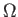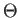Next: Boolean Functions Up: Graph Properties Previous: Bipartiteness   Contents

# No Quantum Extension of the Aanderaa-Karp-Rosenberg Conjecture

The Aanderaa-Karp-Rosenberg conjecture states that all non-trivial monotone graph properties are evasive in the classical deterministic setting. A natural extension of the Aanderaa-Karp-Rosenberg Conjecture to quantum computing would conjecture all non-trivial monotone graph properties have the same query complexity, or at least the same asymptotic query complexity in the bounded error setting. To see that no such extension can hold, observe that the following non-trivial monotone graph properties can be determined by running the algorithms for OR, AND, and MAJORITY respectively:

1. Does the graph have at least 1 edge?
2. Is the graph complete?
3. Does the graph have more than half of all possible edges?

Beals et al. provide an O(V) oracle query algorithm for computing the AND or OR of an O(V2) bit oracle string in the bounded error setting , and we have a lower bound of(V) oracle queries required to compute them from Section 2.6. We have a lower bound of(V2) oracle queries required to compute the MAJORITY of an O(V2) bit oracle string from Section 2.6. Thus some decision problems for non-trivial monotone graph properties require(V) oracle queries and others(V2) in the bounded error setting, and there is no extension of the Aanderaa-Karp-Rosenberg conjecture to the quantum bounded error setting. This does not come as a complete surprise, as there are quadratic gaps known between the classically evasive symmetric functions from Chapter 2 in the quantum bounded error setting. More disappointing is that there are graph properties for which the quantum bounded error setting can provide only constant speedup over the classical case.Next: Boolean Functions Up: Graph Properties Previous: Bipartiteness   Contents
Matthew Hayward Lower Query Bounds in the Quantum Oracle Model GitHub Repository